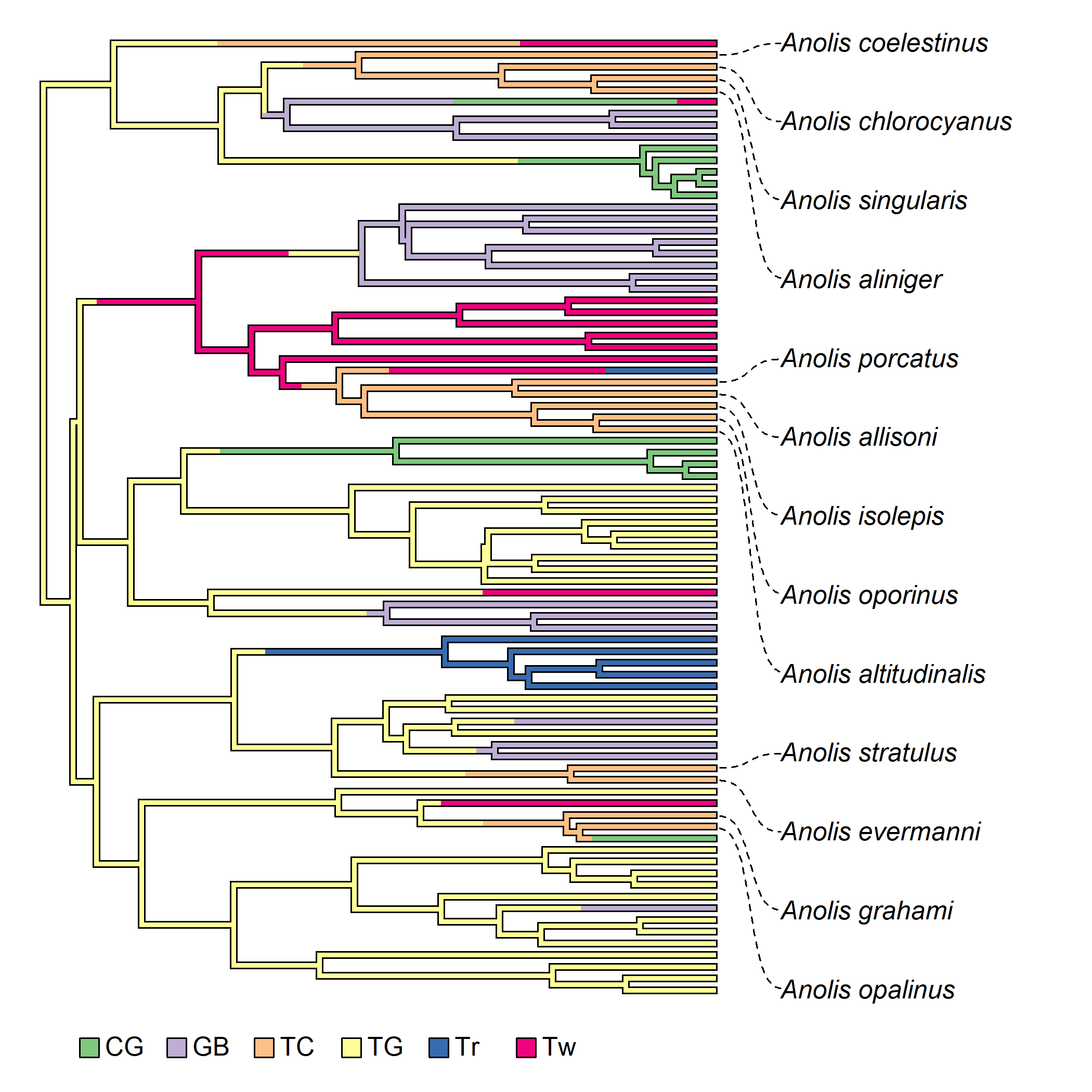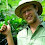## Monday, June 10, 2019

### Using `linklabels` to plot tip labels for only taxa with a particular state of a discrete character

As I work (more or less alphabetically) through the >200 different functions of the phytools package, doing updates & various other maintenance before submitting a new CRAN version (hopefully by the end of the week), I stumbled on the function `linklabels`. This is a neat function that can be used to annotate a plotted phylogeny. Here is a quick demo in which I plot only tip labels (with linking lines) for taxa that have a particular value for a phenotypic character - in this case the Anolis ecomorph state of `"TC"` ('trunk-crown'):

``````library(phytools)
data(anoletree)
ecomorph<-as.factor(getStates(anoletree,"tips"))
TC<-names(ecomorph)[which(ecomorph=="TC")]
library(RColorBrewer)
cols<-setNames(brewer.pal(n=length(levels(ecomorph)),
"Accent"),levels(ecomorph))
## this is to plot an outline for my 'simmap' tree
plotTree(anoletree,xlim=c(0,9),
ylim=c(-4,Ntip(anoletree)),
ftype="off",lwd=5)
plot(anoletree,cols,xlim=c(0,9),
ylim=c(-4,Ntip(anoletree)),
x=0.5,y=-4,vertical=FALSE)
tips<-sapply(TC,function(x,y) which(y==x),
y=anoletree\$tip.label) ## match node numbers
``````I think that looks kind of cool.

1.1.